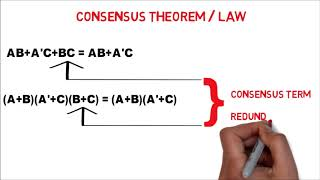# Consensus Theorem

In 1854, George Boole, an English mathematician, proposed variable-based math for emblematically addressing issues in rationale so they might be broken down numerically. The numerical frameworks established upon crafted by Boole are called Boolean polynomial math in his honor. The use of Boolean variable-based math for specific designing issues was presented in 1938 by C.E. Shannon. For the proper meaning of Boolean variable-based math, we will utilize the proposes figured out by E.V. Huntington in 1904.

A few of them we have already discussed in the previous article. In this article, we will be focusing only upon the consensus theorem as this particular theorem is mostly asked during the interviews of many Electronics companies for VLSI Design , FPGA Design Engineer , RTL Design Engineer, SOC Verification Engineer , Physical Design Engineer , STA Engineer , Emulation Engineer role in many product and service based companies such Qualcomm , Intel , AMD , Microchip , Micron Technology, Truechip Solution , Wipro , HCL , LnT and many other popular companies .

So I suggest you all to understand this topic very clearly.

In simplification of Boolean expression, an expression of the form AB+ A’C+ BC, the term BC is redundant and can be eliminated to form the equivalent expression AB+A’C.

The theorem used for this simplification is known as consensus theorem and is stated as,

The consensus theorem -1 :
The consensus theorem can be stated as follows: XY + X’Z + YZ = XY + X’Z
Proof:
XY + X’Z + YZ
XY + X’Z + (X + X’)YZ
XY + X’Z + XYZ + X’YZ
(XY + XYZ) + (X’Z + X’YZ)
XY(1 + Z) + X’Z(1 + Y)
XY + X’Z

The consensus theorem – 2:
Example : Simplify this expression X’Y’ + XZ + YZ’ + Y’Z + XY
X’Y’ + XZ + YZ’ + Y’Z + XY
Ans:X’Y’ + XZ + YZ

The consensus theorem – 3 :
The dual form of the consensus theorem is(X + Y)(X’ + Z)(Y + Z) = (X + Y)(X’ + Z)
Example : (A + B + C’)(A + B + D’)(B + C + D’)
The Consensus of (A + B + C’) and (B + C + D’) is(A + B + D’)
Hence, we can eliminate the consensus term
Answer : (A + B + C’)(A + B + D’) is the Final output

So guys based on the above theorem I have given two assignment below to check your understanding . I suggest you all to try it by yourself and post your answer in the discussion box.

Assignment-1
Simplify each of the following expressions using the consensus theorem:
BC’D’ + ABC’ + AC’D + AB’D + A’BD’
A’C’ + ACD + AC’D + AB’C

The US Hits China With a Huge Microchip Bill FPGA Design Engineer Interview Questions Semiconductor Industry the huge break through